# Primary School Math Question Divides The Internet, Even Calculators Disagree On The Answer

## Primary School Math Question Divides Twitter As Users Defend 2 Different Answers

8 ÷ 2(2+2) = ?

It’s because this is the most viral math equation floating around the Internet right now.

Many netizens have been scrambling to explain their answers to this baffling mathematical question.

On Monday (29 Jul), Twitter user @pjmdoll tweeted this million dollar equation, asking netizens to solve it.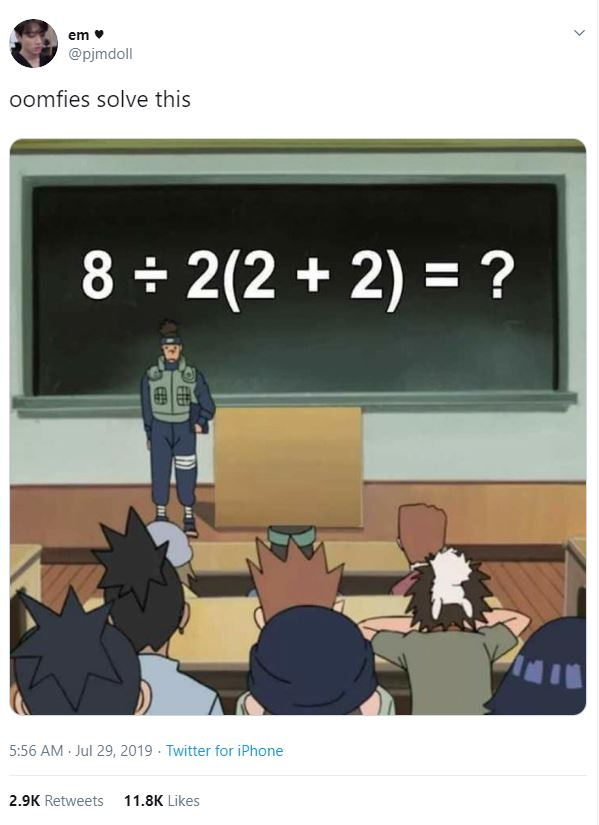• Pinterest
• reddit
Source

But no one can decide whether the answer is 1 or 16.

The trending tweet has since amassed almost 3,000 retweets and over 11,800 likes.

### Calculators can’t decide on math question too

You know the problem is real when calculators disagree with the final answer too.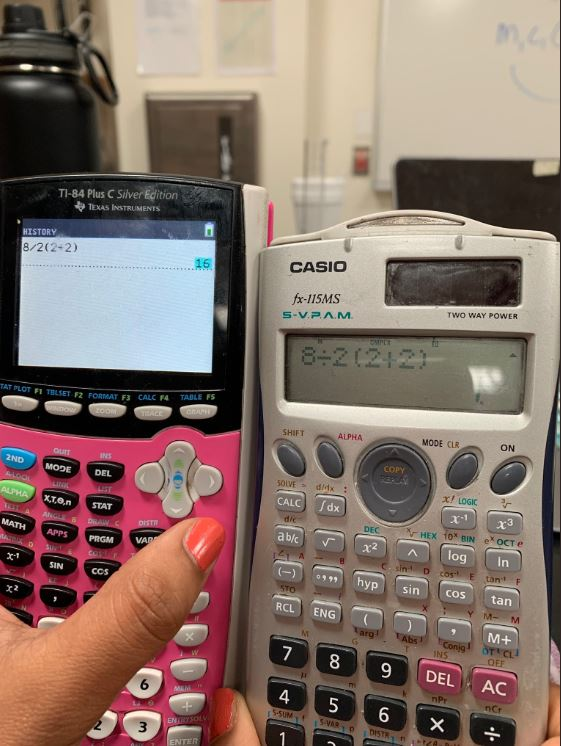• Pinterest
• reddit
Source

After doing some quick maths yourself, what did you get?

If you got 1 and feel pretty confident about it, just remember there’s a whole legion of people on the other side defending the answer 16 — and vice versa.

Let’s have a look at what Twitter users have to say.

This user uses a screenshot of her formula to justify why the answer is 16.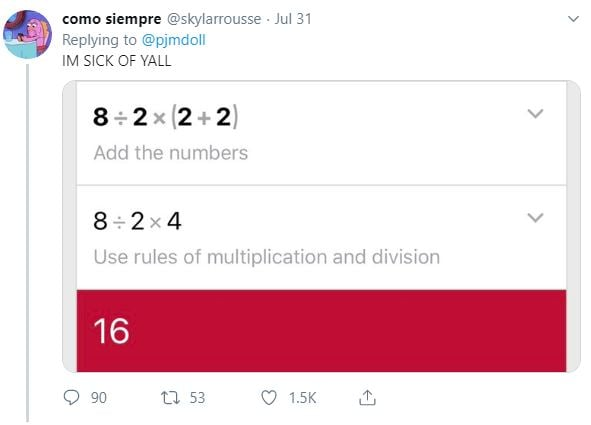• Pinterest
• reddit
Source

Same goes for this user, who’s adamant that it’s 16.• Pinterest
• reddit
Source

Now it’s time for those rallying behind team “1” to shine, like this user who flexes her 2 math degrees and says it’s 1.• Pinterest
• reddit
Source

Here’s how the calculation is done, as shown by this user, to get 1 as the answer.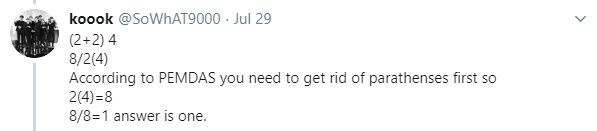• Pinterest
• reddit
Source

### Answer depends on order of operations

After polling our readers on Telegram, we received 1,217 votes at the time of writing, with 70% voters choosing “1”.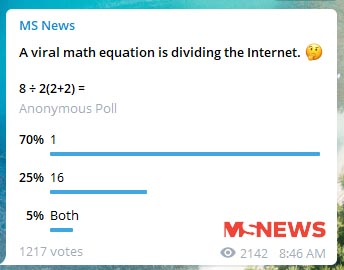• Pinterest
• reddit

We decided to do further digging – and asking our fellow friends with engineering degrees – to get to the bottom of this.

Apparently, people are getting different answers because the answer lies in the way you solve it. And this depends on which part of the world you learnt mathematics.

Essentially, what this viral question reveals is that there are parts of the world where people are taught the BODMAS method, and the other part of the world the PEMDAS method.

If you’re lost, here are what the letters stand for.

BODMAS:

• Brackets
• Orders
• Division
• Multiplication
• Subtraction

Applying this rule, people get 1 because they solve everything by removing brackets first. As such, 8 ÷ 2(2+2) = 8 ÷ 2(4) = 8 ÷ 8 = 1

PEMDAS:

• Parenthesis
• Exponents
• Multiplication
• Division
• Subtraction

With this rule, where multiplication and division take equal priority after parenthesis, people get 16 because they start calculating from left to right once they’ve solved 2+2 in the parenthesis.

Hence, 8 ÷ 2 x (2+2) = 8 ÷ 2 x 4 = 4 x 4 = 16.

### It’s not you, it’s where you learnt math

After learning this difference in order of operations, it seems that there may be 2 correct answers to this primary school math question.

While some have relented that the problem lies with the way the equation was written, some refuse to believe that both answers are correct.

What do you make of this? Share your thoughts with us in the comments below.